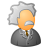# HOW TO VALUE ROW COUNT?

3706
4
03-10-2015 08:19 AMby
New Contributor II

I Have A Fc With A Field Which Contain
1,2,3 Value.

I Need To Write A Code That Give Me
The Total Count For Each Value:

1=29

2=52

3=57

this is the code:

print "start"

import arcpy

1. arcpy.env.overwriteOutput = 1

fc =r"D:\AVI_DAVID\zevel\zevel.gdb\Export_Output_miv_cur"

lst_field =arcpy.ListFields(fc,"USAGE")      # usage is the field

for f in lst_field:

print f.name

DO I NEED A SEARCHCURSOR? OR
UPDATECURSOR?

AVI

1 Solution

Accepted SolutionsbyMVP Esteemed Contributor

See Summary Statistics if you have an Advanced license, check out the Frequency tool while you are there

4 RepliesbyMVP Esteemed Contributor

See Summary Statistics if you have an Advanced license, check out the Frequency tool while you are thereby
New Contributor II

Frequency Tool Make A Tbl(Output) Which Is Good, But I Need The Result Inside
The Code

AVIbyMVP Esteemed Contributor

I am not sure what you mean by the result in the code...do you need the code to perform the task?  If so, use the snippet from the help files or from the Results window after a successful run of the tool.

If need the actual results as a variable for further use in your code, you will have to use an arcpy.da searchcursor and I use numpy to do the grunt work.  totally untested, but the sequence would be

After the help files on arcpy.da.searchcursor

Try this from the command line in your IDE

```import arcpy
input_shp = 'c:/yourpath/yourfilename.shp'
input_field = 'num_field'   # an integer field as an example
values = [row for row in arcpy.da.SearchCursor(input_shp, input_field)]
uniqueValues = set(values)  #from the help files```

that will get you the values in the field, and their unique classes:

I tend to switch over to numpy if I already haven't converted the whole file to an array already.

```>>> import numpy as np
>>> data = [1,2,2,2,1,1,1,3,3,2,3,3,4]
>>> classes = np.unique(data)
>>> freq = np.histogram(data,classes)
>>>
>>> classes
array([1, 2, 3, 4])
>>> freq
(array([4, 4, 5]), array([1, 2, 3, 4]))
>>>```

which as you can see, there are 4 1's, four 2s, and 5 > 3

if you want an exact count, then you can play around with the bins as in this example

```>>> data = [1,2,2,2,1,1,1,3,3,2,3,3,4]
>>> classes = np.unique(data)
>>> classes = np.unique(data).tolist()
>>> classes.append(classes[-1]+1)
>>> classes
[1, 2, 3, 4, 5]
>>> freq = np.histogram(data,classes)
>>> freq
(array([4, 4, 4, 1]), array([1, 2, 3, 4, 5]))
>>>```

Of course, you can incorporate the command line stuff in your codeby
New Contributor II

Do the same as Frequency( Frequency better)...